## Monday, 25 April 2011

### Auto bed leveling

One thing I find tedious is leveling the bed of my machines, so I decided to make use of the Z-probe on HydraRaptor to measure the incline of the bed and compensate for it in software.

First I had to increase the resolution of my Z axis. When I first built the machine I did not realise that I would need fine resolution on Z, so I used an old 24V unipolar motor that I had in my junk collection. With half stepping it gave me 0.05mm resolution. I thought that it was a 200 step motor and that the lead screw had a pitch of 20mm. It turns out it must have been a 250 step motor because the pitch is actually 25mm. I replaced it with a Keling KL23H251-24-8B NEMA23 left over from my Darwin and I now drive it with a x10 microstepping controller the same as I use on X and Y. That gives me a resolution of 0.0125mm and also makes it the fastest axis on my machine. It can easily do 150 mm/s but seeing the nozzle approaching the bed at that speed and then stopping within 0.3mm is very unnerving, so I limit the speed to 50mm/s!

I no longer need the heat sink and fan because the new motor is more efficient and is directly mounted on the axis, which takes the heat away.

I use 6mm aluminium tooling plate so I make the assumption that the bed is a flat plane (rotated slightly around the X and Y axes and offset a little in Z). That means I only need to measure the height at three arbitrary points in order to characterise that plane. I then use the method here to calculate the equation of the plane in the form ax + by +cz +d = 0. The method puts two vectors through the three points and takes the cross product to get a vector at right angles to both of them. That is the normal to the plane and its components are the coefficients a, b and c. Substituting the first point into the equation gives d.

It is important that the three points are ordered anti-clockwise, otherwise the normal vector would point downwards and the machine would try to build the object upside down under the surface of the bed!

Given the bed's plane I then have to make the model coordinates relative to that inclined plane and transform them to the coordinate system of the machine. To do that I calculate two orthonormal basis vectors on the plane using it's equation and use the normalised normal vector for the third. I then multiply the model coordinates by those vectors and add the origin to find where they are in the machine's coordinates. Here is the Python code I used: -

class Plane:
"A plane in 3D."

def __init__(self, p0, p1, p2):
"Construct from three anti-clockwise points"
#
# Calcluate the normal vector
#
v1 = p1.minus(p0)
v2 = p2.minus(p0)
normal = v1.cross(v2)
if normal.z < 0:
raise Exception, "Probe points must be anti-clockwise"
#
# Coefficients of the plane equation ax + by + cz + d = 0
#
a = normal.x
b = normal.y
c = normal.z
d = -a * p0.x -b * p0.y -c * p0.z
#
# Generate three basis vectors aligned with the plane
#
self.origin = vector( 0, 0, -d / c)

self.k = normal                      # k axis is simply the normalised normal
self.k.normalize()

px = vector( 1.0, 0.0, -(a + d) / c) # an arbitrary point on the x axis: x = 1, y = 0
self.i = px.minus(self.origin)       # find direction to it from origin
self.i.normalize()                   # make a unit vector

self.j = self.k.cross(self.i)        # make a third vector mutually at right angles to the other two
self.j.normalize()                   # make a unit vector, probably is already

def transform(self, p):
"Transform a point to be relative to the plane"
return self.origin.plus(self.i.times(p.x)).plus(self.j.times(p.y)).plus(self.k.times(p.z))


To test the principal I put a 1mm thick washer under one corner support of the bed to give it an extreme slant compared to normal misalignment. I then built a 100 x 100 x 5mm cube with 0.35mm layers. This would normally be impossible without the bed being level to a small proportion of the layer height. The result was that it came out fine.

As the nozzle traverses the object in XY the Z axis moves a few microsteps. It is barely visible but I can hear it and feel it if I hold the stepper motor shaft. The object is built perpendicular to the plane of the bed, so the sides are very slightly slanted with respect to the machine axis and the nozzle. I am not sure how well it would work on Mendel as the z-axis is geared down so much. It would probably still work as the movement required is so small when the bed is reasonably level. I can't test it as there isn't room for a z-probe on my carriage due to the large heat sink.

1.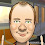...and here I thought you were going to have the z-probe measure three points, then tell you exactly how many turns to make (in which direction) on each screw! It seems both ways would be nice to have - making it actually level (with continuous z-probe re-checking), and software compensation all the time. Now I want a z-probe! :)

2.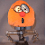Well the problem is I don't have any screw adjustment on HydraRaptor, I have to shim it, and being made of wood it can vary a little.

My Mendel is more stable but it did change when I took it on a trip that involved an accidental detour down a one way cobbled street!

3.What you propose would be very easy. Simply put the XY coordinates of the supports into the plane equation to get the Z value. Subtract the Z value of the plane's origin and that divide that by the screw pitch.

4.Can you help me to write a small script to modify a gcode text file with three z-probe measurements?

inputs:gcode text file and zprobe measuremnts
output: modified gcode

5.I agree with Nuri, that would certainly be handy. :-)

6.Yet another neat solution to a problem.

7.Nuri,
I suggest you borrow the g-code import and export functions from Skeinforge and put each point through my Plane.transform function.

Ideally it should be done by the host program so it can probe before every build.

8.Thanks nophead, very interesting. I've just spent the last week doing something very similar - mapping out the bed on my Mendel. I found that the springs on the Mendel are slightly distorting the bed, so I've done away with them (see blog for details).

My next step was to develop some code to modify the GCode to account for any deviation of the bed, but it looks like I can just run my data through your code. Thanks for the investigation, very interesting and thorough as always.

9.Could you not eliminate the slant of the sides by offsetting each layer in XY to correct it?

10.Yes that is exactly what I am doing. The sides are slanting with respect to the Z axis but they are perpendicular to the bed, so the object has the correct geometry.

11.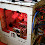Awesome! MBI needs to add this feature.

12.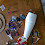What? No one has a dial indicator? I've just picked one up at Harbor Freight for ~\$30.

Method:
Just set up the dial indicator against your build platform. Move your table around under it taking your readings where you like and tweak the table as you go.

Then - I'm anxious to do the nophead math to incorporate into my bot so it will build perpendicular to my slanted x-y plane. That is cool!

13.Tip that I just worked for leveling beds. Suggestion: do the Tip then do Nophead's technique and we should not have any problem with BP tilt.

Use a setting putty, dough or paste (material). I used J-B Weld and took out 1/2 of the grey (hardener) dough to slow the set time. Mix or prepare your material for application.

Take off your build platform (BP). Cover the points where the BP plate touch the support structure with cellophane as a release agent.

Now, put a small pinch of material at each bolt and anywhere else there would be support on the underside of the BP.

Quickly so you have working time with the material replace the BP. Put in the screws and just catch a couple of the nut threads.

Place your recently purchased dial gauge in place to measure the level of your BP.

Move the BP around to the low point and mark on paper the number. Then move BP around under the dial gauge tightening the screws as needed to begin bringing all the points to the number recorded at the first lowest point. After you are done you could have all points on your BP, assuming the platform is flat, within 0.0005 (The accuracy of my dial gauge) of each other.

Let the material set. You can then take off and replace your BP. It will maintain the settings without shim or adjustments.

I actually gave up at 0.001. That is 1/1000th of an inch across a 4" X 4" BP. Kinda ok?

14.Yes but the point is that if the machine is made from wood it varies with the weather and can change noticeably from one build to the next. That is why I probe before every build.

15.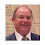I like the python, can you divulge what package you are using for the matrix math?

16.The vec3 class from a very old version of Skeinforge.

17.Why not have the extruder put down a layer of disposable plastic that compensates for for the slope of the bed and makes a level area to build the part on?

18.That is what I used to do when I used rafts, but it wastes machine time and plastic and it wastes my time removing the raft. Also it does not give as smooth a base on the object.

19.hi, ive been trying to piece together an extruder from the junk i have laying around and found a interesting possibility. i gutted an old stepper motor adn found that teh magnet inside has nice teeth with a 2mm gap down the center, so im thinnkig about press fiting this onto my stepper motor shaft, have you seen this before or have anythoughts about it?
thanks

20.I have seen the inside of stepper motors but I don't remember seeing anything that looked like it would grip filament.

21.Commercial CNC Routers use a calibrating block to measure Z height.. It works the way that you have ground connected to the Metal probe of previously measured thickness. Basically you let it close the circuit when the nozzle touches the probe. Then You offset Z by the calibration block thickness. That gives you your Z.height.

22.Does anyone recommend which reprap clone is the best in terms of quality.. most of them I have seen are not very good... alot of these people selling them DO not advertise the end result of the prints or supply really low quality photos... I smell something dodgy in the wind there... nophead's work is excellent shame he does not supply complete exact kits for the same results :(... nophead you should name it the reprap nophead... that way you can show everyone the end result more than the machine itself...

23.What exactly is the .normalize() function doing to the vector to turn it into a unit vector? I'm just trying to figure out the math. Also, in the transform method you are adding each unit vector to the x, y, and z points of point p and then adding that vector to the origin? I assume that you are returning a vector but I do not know how python performs the plus() method, so to me it appears as if you are doing this: (origin+i*px)+(j*py)+(k*pz). The transform method seems pretty simple, couldn't this be run in the firmware for those of us still using gcode?

24.Normalize works out the length of the vector by taking the square root of the sum of each element squared. It then divides each element by that length so the new length is one but the vector points in the same direction.

Yes each point is transformed using the equation above but origin, i, j and k are three element vectors, so each multiply is actually three floating point multiplies and each add is three floating point adds. Floating point operations on an 8 bit micro are very slow because C normally converts all the operands to doubles which are 8 bytes each. I don't know whether that is the case on an ATMEGA but I doubt it will be fast enough for short line segments even with 4 byte floats. Each float multiply would be at least 9 8x8 multiplies and many adds.

The best place to do it is in the host program. It could easily parse the G1 commands and modify them before sending them on to the micro.

1.So, just to see if I understand...when p.x is multiplied by i, it is more like (i.x*p.x, i.y*p.x, i.z*p,x).

2.Yes exactly. A move in the X direction of the model potentially causes a move in all three axes of the machine if the bed is tilted.